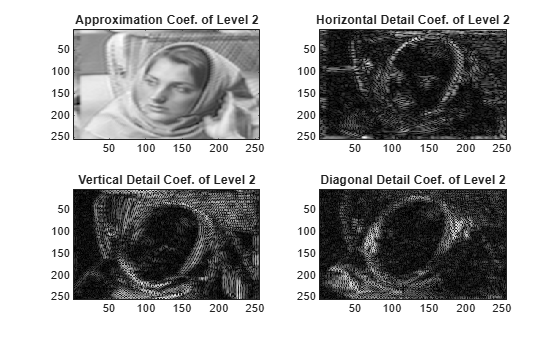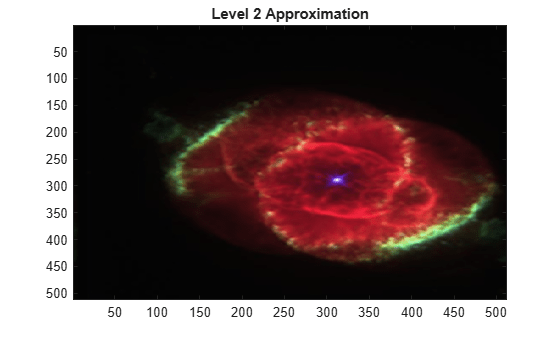# swt2

Discrete stationary wavelet transform 2-D

## Syntax

```SWC = swt2(X,N,'wname') [A,H,V,D] = swt2(X,N,'wname') SWC = swt2(X,N,Lo_D,Hi_D) [A,H,V,D] = swt2(X,N,Lo_D,Hi_D) ```

## Description

`swt2` performs a multilevel 2-D stationary wavelet decomposition using either an orthogonal or a biorthogonal wavelet. Specify the wavelet using its name(`'wname'`, see `wfilters` for more information) or its decomposition filters.

`SWC = swt2(X,N,'wname')` or ```[A,H,V,D] = swt2(X,N,'wname')``` compute the stationary wavelet decomposition of the real-valued 2-D or 3-D matrix `X` at level `N`, using `'wname'`.

If `X` is a 3-D matrix, the third dimension of `X` must equal 3.

`N` must be a strictly positive integer (see `wmaxlev` for more information), and 2N must divide `size(X,1)` and `size(X,2)`.

The dimension of `X` and level `N` determine the dimensions of the outputs.

• If `X` is a 2-D matrix and `N` is greater than 1, the outputs `[A,H,V,D]` are 3-D arrays, which contain the coefficients:

• For `1 `` i ``N`, the output matrix `A(:,:,i)` contains the coefficients of approximation of level `i`.

• The output matrices `H(:,:,i)`, `V(:,:,i)` and `D(:,:,i)` contain the coefficients of details of level `i` (horizontal, vertical, and diagonal):

```SWC = [H(:,:,1:N) ; V(:,:,1:N) ; D(:,:,1:N) ; A(:,:,N)] ```

• If `X` is a 2-D matrix and `N` is equal to 1, the outputs `[A,H,V,D]` are 2-D arrays where `A` contains the approximation coefficients, and `H`, `V`, and `D` contain the horizontal, vertical, and diagonal detail coefficients, respectively.

• If `X` is a 3-D matrix of dimension `m`-by-`n`-by-3, and `N` is greater than 1, the outputs `[A,H,V,D]` are 4-D arrays of dimension `m`-by-`n`-by-3-by-`N`, which contain the coefficients:

• For `1 `` i ``N` and `j = 1, 2, 3`, the output matrix `A(:,:,j,i)` contains the coefficients of approximation of level `i`.

• The output matrices `H(:,:,j,i)`, `V(:,:,j,i)` and `D(:,:,j,i)` contain the coefficients of details of level `i` (horizontal, vertical, and diagonal):

```SWC = [H(:,:,1:3,1:N) ; V(:,:,1:3,1:N) ; D(:,:,1:3,1:N) ; A(:,:,1:3,N)] ```

• If `X` is a 3-D matrix of dimension `m`-by-`n`-by-3, and `N` is equal to 1, the outputs `[A,H,V,D]` are 4-D arrays of dimension `m`-by-`n`-by-1-by-3, which contain the coefficients:

• For `j = 1, 2, 3`, the output matrix `A(:,:,1,j)` contains the approximation coefficients.

• The output matrices `H(:,:,1,j)`, `V(:,:,1,j)` and `D(:,:,1,j)` contain the horizontal, vertical, and diagonal detail coefficients, respectively.

```SWC = [H(:,:,1,1:3) ; V(:,:,1,1:3) ; D(:,:,1,1:3) ; A(:,:,1,1:3)] ```

Note

• `swt2` uses double-precision arithmetic internally and returns double-precision coefficient matrices. `swt2` warns if there is a loss of precision when converting to double.

• To distinguish a single-level decomposition of a truecolor image from a multilevel decomposition of an indexed image, the approximation and detail coefficient arrays of truecolor images are 4-D arrays. See Distinguish Single-Level Truecolor Image from Multilevel Indexed Image Decompositions. Also see examples Stationary Wavelet Transform of an Image and Inverse Stationary Wavelet Transform of an Image.

If an `K`-level decomposition is performed, the dimensions of the `A`, `H`, `V`, and `D` coefficient arrays are `m`-by-`n`-by-3-by-`K`.

If a single-level decomposition is performed, the dimensions of the `A`, `H`, `V`, and `D` coefficient arrays are `m`-by-`n`-by-1-by-3. Since MATLAB® removes singleton last dimensions by default, the third dimension of the coefficient arrays is singleton.

`SWC = swt2(X,N,Lo_D,Hi_D)` or ```[A,H,V,D] = swt2(X,N,Lo_D,Hi_D)```, computes the stationary wavelet decomposition as in the previous syntax, given these filters as input:

• `Lo_D` is the decomposition low-pass filter.

• `Hi_D` is the decomposition high-pass filter.

`Lo_D` and `Hi_D` must be the same length.

Note

`swt2` is defined using periodic extension. The size of the approximation and details coefficients computed at each level equals the size of the input data.

## Examples

collapse all

Extract and display images of the stationary wavelet decomposition level coefficients. First load and display the original image. Then perform the stationary wavelet decomposition of the image at level 2 using `db6`.

```load woman imagesc(X) colormap(map) title('Original')````[ca,chd,cvd,cdd] = swt2(X,2,'db6');`

Extract the Level 1 and Level 2 approximation and detail coefficients from the decomposition.

```A1 = wcodemat(ca(:,:,1),255); H1 = wcodemat(chd(:,:,1),255); V1 = wcodemat(cvd(:,:,1),255); D1 = wcodemat(cdd(:,:,1),255); A2 = wcodemat(ca(:,:,2),255); H2 = wcodemat(chd(:,:,2),255); V2 = wcodemat(cvd(:,:,2),255); D2 = wcodemat(cdd(:,:,2),255);```

Display the approximation and detail coefficients from the two levels.

```subplot(2,2,1) imagesc(A1) title('Approximation Coef. of Level 1') subplot(2,2,2) imagesc(H1) title('Horizontal Detail Coef. of Level 1') subplot(2,2,3) imagesc(V1) title('Vertical Detail Coef. of Level 1') subplot(2,2,4) imagesc(D1) title('Diagonal Detail Coef. of Level 1')``````subplot(2,2,1) imagesc(A2) title('Approximation Coef. of Level 2') subplot(2,2,2) imagesc(H2) title('Horizontal Detail Coef. of Level 2') subplot(2,2,3) imagesc(V2) title('Vertical Detail Coef. of Level 2') subplot(2,2,4) imagesc(D2) title('Diagonal Detail Coef. of Level 2')```In this example you obtain single-level and multilevel stationary wavelet decompositions of a truecolor image. You view results of each decomposition.

Load in a truecolor image. The image is a 3-D array of type `uint8`. Since `swt2` requires the first and second dimensions both be divisible by a power of 2, extract a portion of the image and view it.

```imdata = imread('ngc6543a.jpg'); x = imdata(1:512,1:512,:); imagesc(x)```Obtain the 4-level stationary wavelet decomposition of the image using the `db4` wavelet. Return the approximation coefficients and the horizontal, vertical, and detail coefficients as separate arrays. Note the dimensions of the output arrays.

```[a,h,v,d] = swt2(x,4,'db4'); size(a)```
```ans = 1×4 512 512 3 4 ```
`size(h)`
```ans = 1×4 512 512 3 4 ```
`size(v)`
```ans = 1×4 512 512 3 4 ```
`size(d)`
```ans = 1×4 512 512 3 4 ```

The output arrays are all of type `double`. View the level 2 approximation coefficients. Since the approximation coefficients are of type `double`, cast them as `uint8`, which is the datatype of the image.

```figure imagesc(uint8(a(:,:,:,2))) title('Level 2 Approximation Coefficients')```Reconstruct the image by performing the inverse transform. Compute the difference between the original image and reconstruction. Since the reconstruction is of type `double`, cast the reconstruction as type `uint8` before computing the difference.

```rec = iswt2(a,h,v,d,'db4'); maxdiff = max(abs(uint8(rec(:))-x(:))); disp(['maximum difference = ' num2str(maxdiff)])```
```maximum difference = 0 ```

Obtain the single-level stationary wavelet decomposition of the image using the `db4` wavelet. Return the approximation coefficients and horizontal, vertical, and detail coefficients in separate arrays. Note the dimensions of the output arrays.

```[a,h,v,d] = swt2(x,1,'db4'); size(a)```
```ans = 1×4 512 512 1 3 ```
`size(h)`
```ans = 1×4 512 512 1 3 ```
`size(v)`
```ans = 1×4 512 512 1 3 ```
`size(d)`
```ans = 1×4 512 512 1 3 ```

View the approximation coefficients. To prevent an error when using `imagesc`, squeeze the approximation coefficients array to remove the singleton dimension.

```asqueeze = squeeze(a); size(asqueeze)```
```ans = 1×3 512 512 3 ```
```figure imagesc(uint8(asqueeze)) title('Approximation Coefficients')```Reconstruct the image by performing the inverse transform. Compute the difference between the original image and reconstruction. Since the reconstruction is of type `double`, cast the reconstruction as type `uint8` before computing the difference.

```rec = iswt2(a,h,v,d,'db4'); maxdiff = max(abs(uint8(rec(:))-x(:))); disp(['maximum difference = ' num2str(maxdiff)])```
```maximum difference = 0 ```

This example shows how to reconstruct a truecolor image from a single-level stationary wavelet decomposition using 3-D approximation and detail coefficient arrays.

Load in a truecolor image. The image is a 3-D array of type `uint8`. Since `swt2` requires the first and second dimensions both be divisible by a power of 2, extract a portion of the image and view it.

```imdata = imread('ngc6543a.jpg'); x = imdata(1:512,1:512,:); imagesc(x)```Obtain the single-level stationary wavelet decomposition of the image using the `db4` wavelet. Return the approximation coefficients and horizontal, vertical, and detail coefficients in separate arrays. Note the dimensions of the output arrays.

```[a,h,v,d] = swt2(x,1,'db4'); size(a)```
```ans = 1×4 512 512 1 3 ```
`size(h)`
```ans = 1×4 512 512 1 3 ```
`size(v)`
```ans = 1×4 512 512 1 3 ```
`size(d)`
```ans = 1×4 512 512 1 3 ```

Squeeze the coefficient arrays to remove their singleton dimensions. Note the dimensions of the squeezed arrays.

```asq = squeeze(a); hsq = squeeze(h); vsq = squeeze(v); dsq = squeeze(d); size(asq)```
```ans = 1×3 512 512 3 ```
`size(hsq)`
```ans = 1×3 512 512 3 ```
`size(vsq)`
```ans = 1×3 512 512 3 ```
`size(dsq)`
```ans = 1×3 512 512 3 ```

So that `iswt2` can properly reconstruct the trueimage from the new coefficient arrays, insert a singleton dimension by reshaping the squeezed arrays. Reconstruct the image with the reshaped coefficient arrays.

```a2 = reshape(asq,[512,512,1,3]); h2 = reshape(hsq,[512,512,1,3]); v2 = reshape(vsq,[512,512,1,3]); d2 = reshape(dsq,[512,512,1,3]); rec = iswt2(a2,h2,v2,d2,'db4');```

Compute the difference between the original image and reconstruction. Since the reconstruction is of type `double`, cast the reconstruction as type `uint8` before computing the difference.

```maxdiff = max(abs(uint8(rec(:))-x(:))); disp(['maximum difference = ' num2str(maxdiff)])```
```maximum difference = 0 ```

## Tips

When X represents an indexed image, X is an `m`-by-`n` matrix. If the level of decomposition `N` is greater than 1, the output arrays SWC or cA, cH, cV, and cD are `m`-by-`n`-by-`N` arrays. If the level of decomposition `N` is equal to 1, the output arrays SWC or cA, cH, cV, and cD are `m`-by-`n` arrays.

When X represents a truecolor image, it becomes an `m`-by-`n`-by-3 array. This array is an `m`-by-`n`-by-3 array, where each `m`-by-`n` matrix represents a red, green, or blue color plane concatenated along the third dimension. If the level of decomposition `N` is greater than 1, the output arrays SWC or cA, cH, cV, and cD are `m`-by-`n`-by-3-by-`N`. If the level of decomposition `N` is equal to 1, the output arrays SWC or cA, cH, cV, and cD are `m`-by-`n`-by-1-by-3.

For more information on image formats, see the `image` and `imfinfo` reference pages.

## Algorithms

For images, an algorithm similar to the one-dimensional case is possible for two-dimensional wavelets and scaling functions obtained from one-dimensional ones by tensor product.

This kind of two-dimensional SWT leads to a decomposition of approximation coefficients at level j in four components: the approximation at level j+1, and the details in three orientations (horizontal, vertical, and diagonal).

The following chart describes the basic decomposition step for images:## Compatibility Considerations

expand all

Behavior changed in R2017b

## References

Nason, G.P.; B.W. Silverman (1995), “The stationary wavelet transform and some statistical applications,” Lecture Notes in Statistics, 103, pp. 281–299.

Coifman, R.R.; Donoho, D.L. (1995), “Translation invariant de-noising,” Lecture Notes in Statistics, 103, pp. 125–150.

Pesquet, J.C.; H. Krim, H. Carfatan (1996), “Time-invariant orthonormal wavelet representations,” IEEE Trans. Sign. Proc., vol. 44, 8, pp. 1964–1970.

## Extended Capabilities